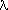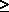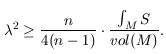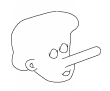# Dirac eigenvalues and total scalar curvature by Bernd Ammann and Christian Bär

Dirac eigenvalues and total scalar curvature (.dvi, .ps, .ps.gz oder .pdf)
J. Geom. Phys. 33, 229-234 (2000)

### Abstract

It has recently been conjectured that the eigenvaluesof the Dirac operator on a closed Riemannian spin manifold M of dimension n3 can be estimated from below by the total scalar curvature:We show by example that such an estimate is impossible. The example contains a very long and thin cylinder and therefore looks like a manifold with a very long nose.58G25

### Keywords

eigenvalues of the Dirac operator, total scalar curvature, Pinocchio metric
Bernd Ammann, 24.6.1999